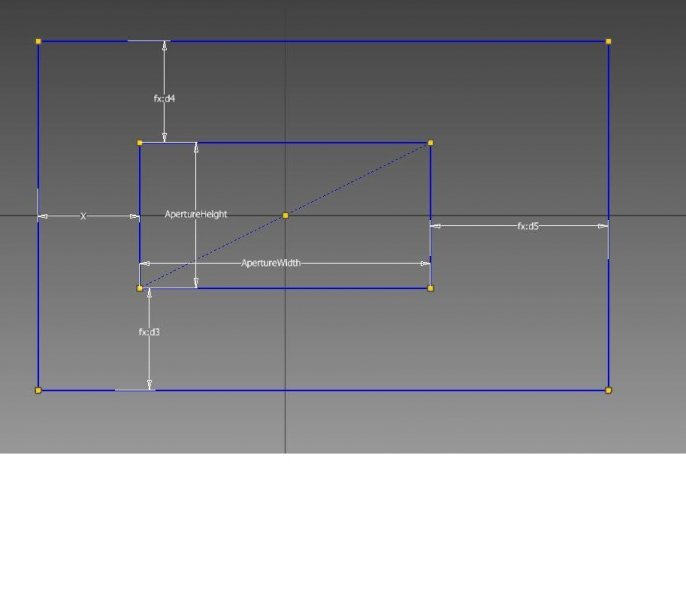# iLogic question

## Recommended PostsGood evening all,

I am trying to make an iPart using iLogic rules and I'm stuck.

The part (put simply) is a solid box with a rectangular hole running right through it, slightly off centre.My sketch includes an 'X' dimension (which is also d3 and d4), that I would like to be determined by what I input as 'ApertureWidth' and 'ApertureHeight'

Below is what I have written as an iLogic rule, however the 'X' dimension is not behaving as I like ie it is always 125mm.

This is my first attempt at iLogic so I'm sure it's something simple but I can't see it.

Any help appreciatedIf ApertureHeight=100 AndAlso ApertureHeight

If ApertureHeight=275 AndAlso ApertureHeight

If ApertureHeight=1025 AndAlso ApertureHeight

If ApertureHeight=1525 AndAlso ApertureHeight

If ApertureHeight=2025 AndAlso ApertureHeight

If ApertureHeight=2525 AndAlso ApertureHeight

If ApertureHeight>=75 And ApertureWidth>=100 AndAlso ApertureHeight

If ApertureHeight>=75 And ApertureWidth>=275 AndAlso ApertureHeight

If ApertureHeight>=75 And ApertureWidth>=1025 AndAlso ApertureHeight

If ApertureHeight>=75 And ApertureWidth>=1525 AndAlso ApertureHeight

If ApertureHeight>=75 And ApertureWidth>=2025 AndAlso ApertureHeight

If ApertureHeight>=75 And ApertureWidth>=2525 AndAlso ApertureHeight

##### Share on other sitesHi,

I have tried to learn ILogic, I will see if I can help you.

##### Share on other sitesHi,

I have tried to learn ILogic, I will see if I can help you.

Hi mate, I know it has been a while, been very busy at work recently.

I have attached the ipt and as you can see I'm not getting far

Signature i-Part [STAINLESS].ipt

Many thanks

##### Share on other sitesI think I've sorted it out now after all, I was missing all the " speech marks "

If ApertureHeight >= "75" And ApertureWidth >= "100" And ApertureWidth

X= "125"

End If

If ApertureHeight >= "75" And ApertureWidth>= "275" And ApertureWidth

X= "135"

End If

If ApertureHeight >= "75" And ApertureWidth >= "1025" And ApertureWidth

X= "145"

End If

If ApertureHeight >= "75" And ApertureWidth >= "1525" And ApertureWidth

X= "155"

End If

If ApertureHeight >= "75" And ApertureWidth>= "2025" And ApertureWidth

X= "165"

End If

If ApertureHeight >= "75" And ApertureWidth >= "2525" And ApertureWidth

X= "175"

End If

If ApertureHeight = "50" And ApertureWidth >= "100" And ApertureWidth

X= "137.5"

End If

If ApertureHeight = "50" And ApertureWidth>= "275" And ApertureWidth

X= "137.5"

End If

If ApertureHeight = "75" And ApertureWidth >= "1025" And ApertureWidth

X= "145"

End If

If ApertureHeight = "75" And ApertureWidth >= "1525" And ApertureWidth

X= "155"

End If

If ApertureHeight = "75" And ApertureWidth>= "2025" And ApertureWidth

X= "165"

End If

If ApertureHeight = "75" And ApertureWidth >= "2525" And ApertureWidth

X= "175"

End If

##### Share on other sitesI did something almost similar to your drawing with holes, I think you can use Select Case as you can see in this topic:

## Join the conversation

You can post now and register later. If you have an account, sign in now to post with your account.
Note: Your post will require moderator approval before it will be visible.×   Pasted as rich text.   Paste as plain text instead

Only 75 emoji are allowed.